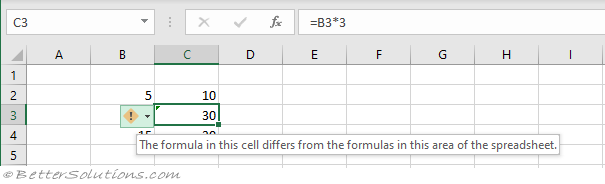# Inconsistent Formula

This rule will identify cells containing formulas that are inconsistent with other formulas in the same region.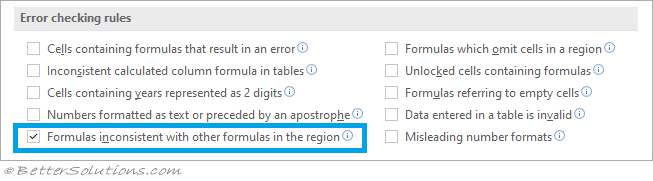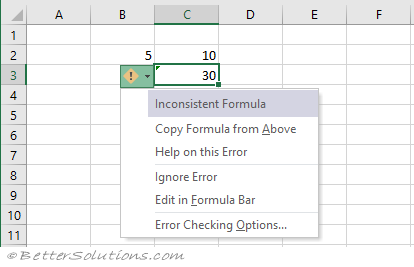Copy Formula From Above / Left - Updates the formula so that it's consistent with the surrounding cells.
Help on this Error - Displays the Help task pane.
Ignore Error - This removes the green triangle from the cell and the cell is no longer considered an error.
Edit in Formula Bar - Places the cursor into the Formula bar so it can be edited.
Error Checking Options - Displays the Options, Formulas Tab.

### Example

When a formula does not match the pattern of other formulas near it.
Enter the values 5, 10 and 15 into cells "B2", "B3" and "B4".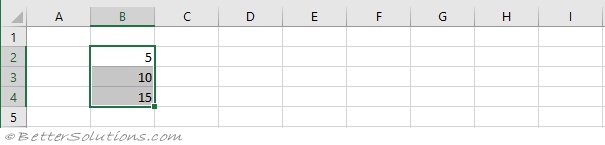Enter the formula "=B2*2" into cell "C2".
Enter the formula "=B3*2" into cell "C3".
Enter the formula "=B4*2" into cell "C4".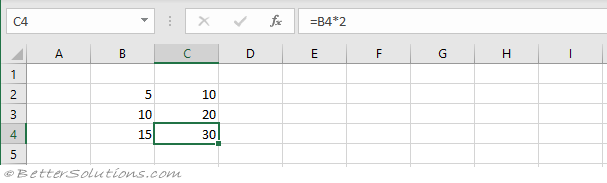Change the formula in cell "C3" to be "=B3*3"